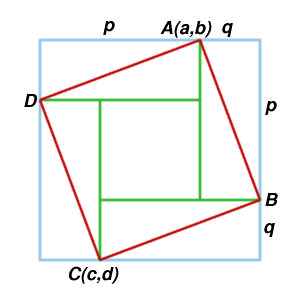#### You may also like### Fitting In

The largest square which fits into a circle is ABCD and EFGH is a square with G and H on the line CD and E and F on the circumference of the circle. Show that AB = 5EF. Similarly the largest equilateral triangle which fits into a circle is LMN and PQR is an equilateral triangle with P and Q on the line LM and R on the circumference of the circle. Show that LM = 3PQ### Pinned Squares

What is the total number of squares that can be made on a 5 by 5 geoboard?### Zig Zag

Four identical right angled triangles are drawn on the sides of a square. Two face out, two face in. Why do the four vertices marked with dots lie on one line?

# Just Opposite

##### Age 14 to 16Challenge Level

Just using coordinates and the clue in the original diagram there is a short and sweet solution sent in by Jo from Leeds.

By concentrating on the geometry, she made the algebra very simple. With the clue that $p-q = a-c$ and $p+q=b-d$ she was able to find the area of the square $ABCD$:

If the vertices were $(6,7)$ and $(3,2)$, the surrounding square would have side $(7-2)$.

The inner square would have a side of $6-3 =3$.
The area of the tilted square is therefore $5 \times 5 - \frac{(5 \times 5)-(3 \times 3)}{2} =17$ sq units and this can be generalised.

In the algebraic case.

Area $= (b-d)^2- \frac{((b-d)^2- (a-c)^2)}{2}$Michael Gray from Madras College solved the first part using vectors.

The midpoint of the square is given by $M=((a+c)/2, (b+d)/2)$. Hence
\begin{eqnarray} \mathbf{CM} &=& \vec{m} - \vec{c} \\ &=& \frac{1}{2} {a-c \choose b-d} \end{eqnarray}
The vector $\mathbf{MD}$ is the vector $\mathbf{CM}$ rotated by $90^o$ anticlockwise and so:
\begin{eqnarray} \mathbf{MD} &=& \frac{1}{2} {-(b-d) \choose a-c} \\ \mathbf{OD} &=& \mathbf{OM} + \mathbf{MD} \\ &=& \frac{1}{2} {a+c \choose b+d} + \frac{1}{2} {-(b-d) \choose a-c} \\ &=& \frac{1}{2} {a-b+c+d \choose a+b-c+d} \end{eqnarray}
and this gives the coordinates of the point $D$. The vector $\mathbf{OB}$, giving the coordinates of $B$, is found in a similar way $$\mathbf{OB} = \frac{1}{2} {a+b+c-d \choose -a+b+c+d}.$$